Disclosure: This post may contain affiliate links, meaning when you click the links and make a purchase, we receive a commission.

# Effective Interest Method of Amortization in Excel

Effective Interest Method of Amortization in Excel (for bonds sold on discount)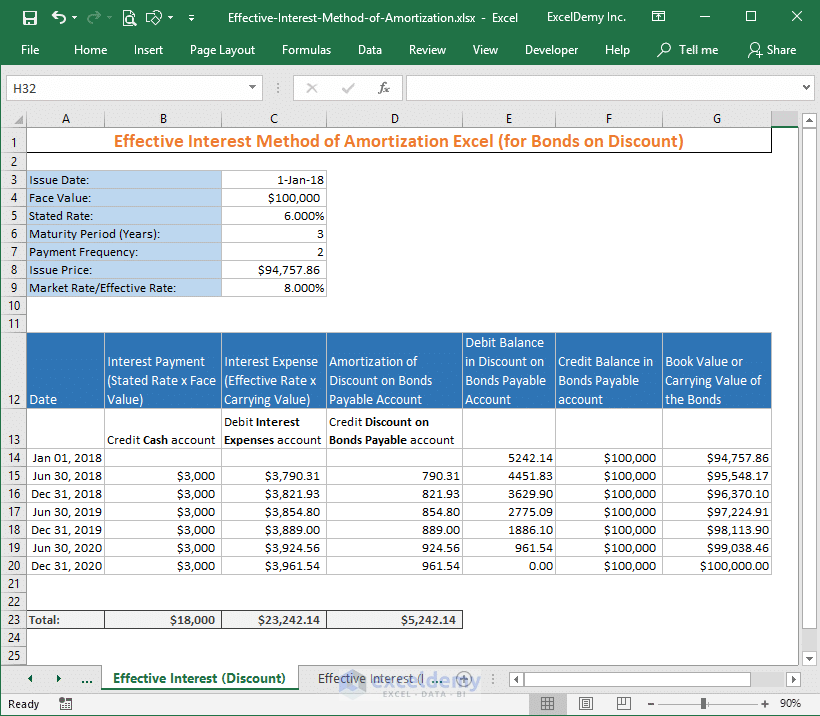Effective Interest Method of Amortization in Excel (for bonds sold on premium)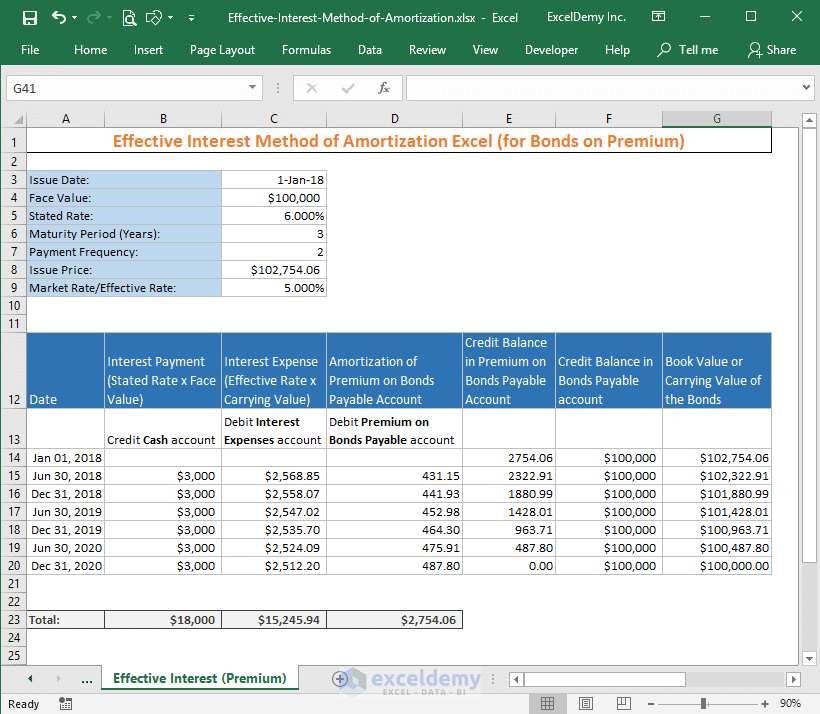In this blog post, I will discuss the effective interest method of amortization in Excel. I will cover the method for both types of bonds:

• Bonds sold on discount
• And bonds sold on premium

## Effective Interest Method of Amortization in Excel (For Bonds Sold on Discount)

Say, your company issued a bond with the following details:

• Issue Date: 1st Jan 2018
• Face Value: \$100,000
• Stated Rate/Nominal Rate/Coupon Rate/APR: 6%
• Market Rate / Effective Annual Interest Rate: 8%
• Maturity Period: 3 years
• Interest Payment Frequency: Semi-annually
• Issue Price: \$94,757.86 (the bond is selling on discount)

You are the accountant of this company and your job is to maintain the accounts for this bond.

How will you do that?

### Step 1: Recording the transactions (Journal Entry)

This is how you will record the transactions that happened on the date 1st Jan 2018.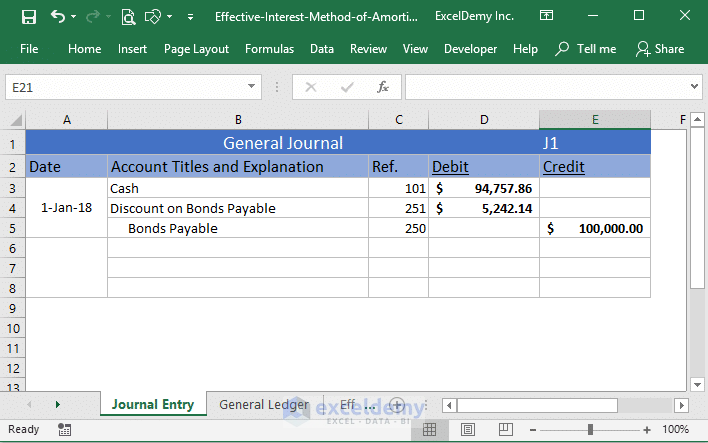Your company has received a cash of amount \$94,757.86. Debits increase assets: so, debit Cash \$94,757.86.

Your company has to pay off the \$100,000 (face value of the bond) after 3 years (as the maturity of the bond is 3 years). So, \$100,000 is credited to the account Bonds Payable.

If you did forget the basics of debit and credit analysis, I want to give you a reminder with the following image.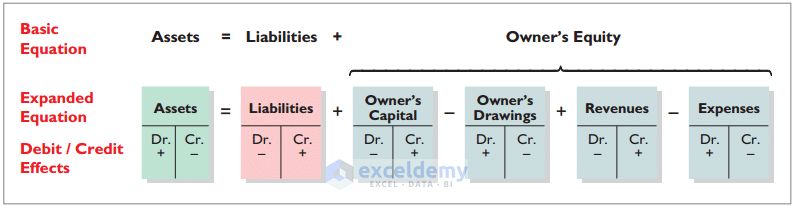You see from the above image, credits increase liability. So, credit Bonds Payable \$100,000.

Now let’s deal with the Discount on Bonds Payable account. This account is created to adjust the discounted amount of the bond.

It is a liability account and we know that debits decrease a liability account (above image). But debits increase this Discount on Bonds Payable account. This is why it is called a contra account. So, debit Discount on Bonds Payable \$5242.14.

### Step 2: Posting

You have posted the above entries in their own accounts and you will get something like the following image.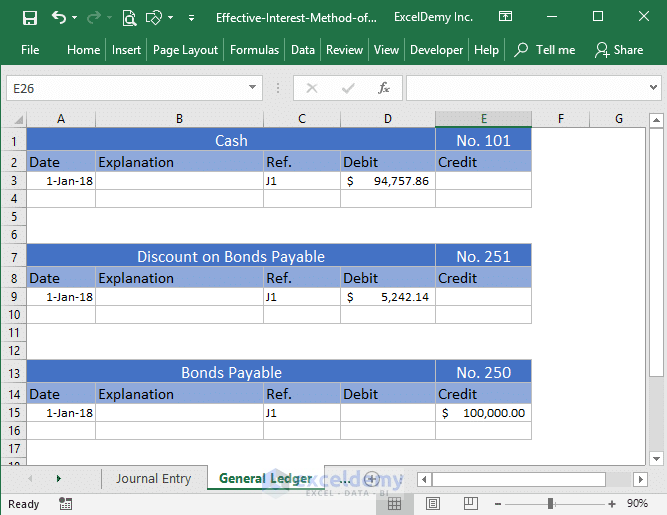### Step 3: Calculating the carrying value of the bond

To calculate the carrying/book value of this bond, you have to subtract the discounted amount from the bond’s face value.

So, on 1st Jan, 2018, the bond’s book value/carrying value = \$100,000 – \$5,242.14 = \$94,757.86.

But over the next 3 years (the maturity period of the bond), this book value will be adjusted in such a way that it will be \$100,000 at the end of the maturity period of the bond.

This slow adjustment (also called amortization) of the book value to its face value can be done in two ways:

• Straight-line Method of Amortization (will discuss it in another article)
• Effective Interest Rate Method of Amortization

Before showing the effective interest rate method of amortization, I want to show some more transactions.

On 30th June 2018, your company is going to pay the bondholder his first semi-annual interest (\$100,000 x 3% = \$3000).

But the real Interest Expense = The book value of the bond x (market rate / 2) = \$94757.86 x (8%/2) = \$3790.31.

Debits increase the expense account. So, I have debited the Interest Expense account (following image) with \$3790.31.

Your company has paid \$3000 cash to the bondholder and credits decrease cash (asset) account. So, credit Cash \$3000.

Discounts on Bonds Payable account is credited by 790.31\$. When liabilities decrease, it goes under the debit column. But as this is a contra account, when liabilities decrease it goes under the credit column.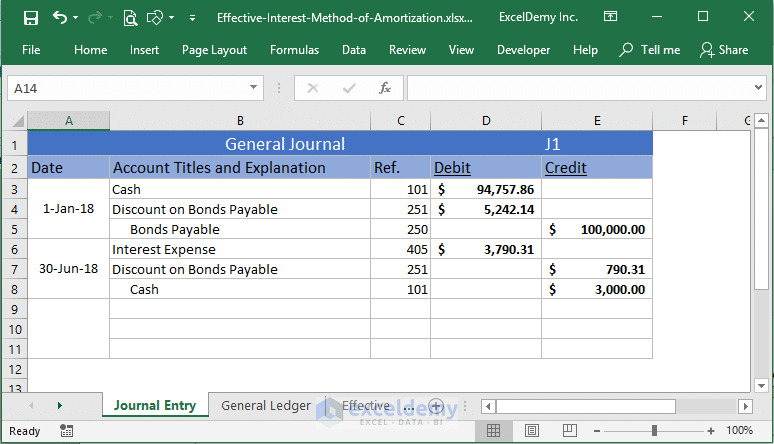And this is my General Ledger now (below image).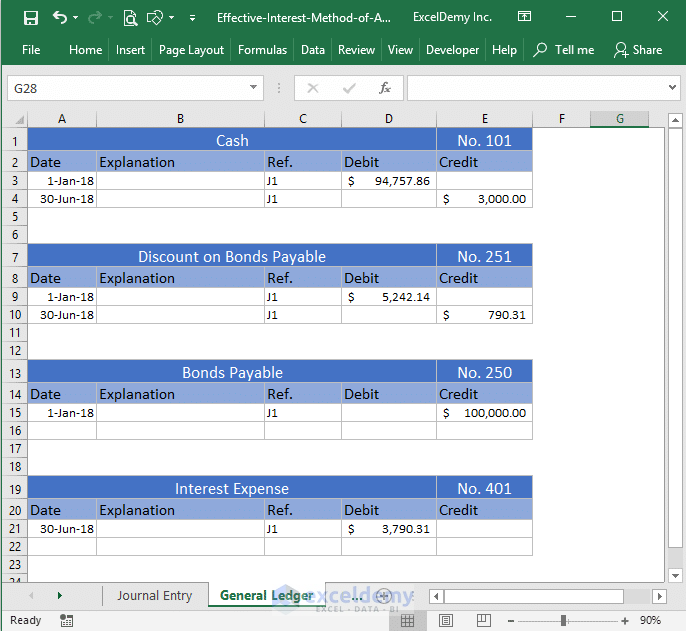You see that we have added a new account (Interest Expense) in our General Ledger now. Why did we add this Interest Expense account? Are the company paying any interest to the bondholder?

The company is paying (every 6 months): Face Value x (Nominal Interest Rate/2) = 100,000 x (6%/2) = \$3000.

But the bond is discounted in such a way that its true return will be 8% every year (the market rate). So, there is a gap between the genuine cost of the fund and the given interest payments. In the Interest Expense account, I have entered the true cost of the fund as \$94757.86 x (8%/2) = \$3790.31.

The difference between the true cost and given interest payment (semi-annually) = \$3790.31 – \$3000 = \$790.31.

This difference (\$790.31) is credited in the Discount on Bonds Payable account.

#### Carrying Value of the Bond

Now let’s think about a virtual account where we shall keep the calculations of the carrying value (book value) of the bond.

The value on the basis of which we calculate the true cost of the fund (that we got from selling a bond) is called the carrying value.

On the issue date of the bond, our bond’s book value (carrying value) was \$94757.86 (issue price).

After 6 months, the book value of the bond will be: \$94757.86 + \$790.31 = \$95,548.17.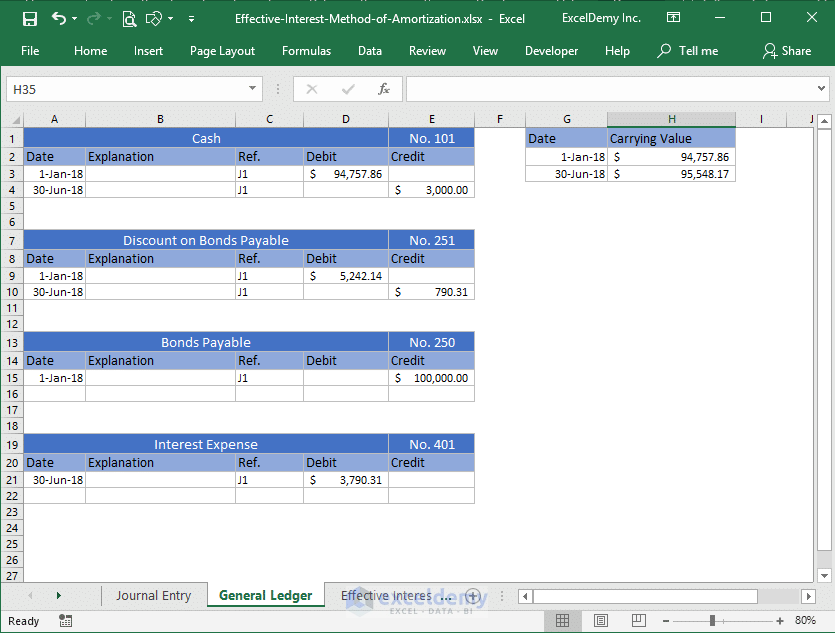How?

Our true cost of the fund was \$3790.31 but we paid \$3000 to the bondholder. The remaining \$790.31 was not paid. As \$790.13 was not paid, the bondholder will get the interest for this unpaid amount at the market rate (8%).

So, after 6 months, the bond’s carrying value will be \$95,548.17.

### Step 4: Let’ check out another transaction

Check out the following journal entry on 31st Dec 2018.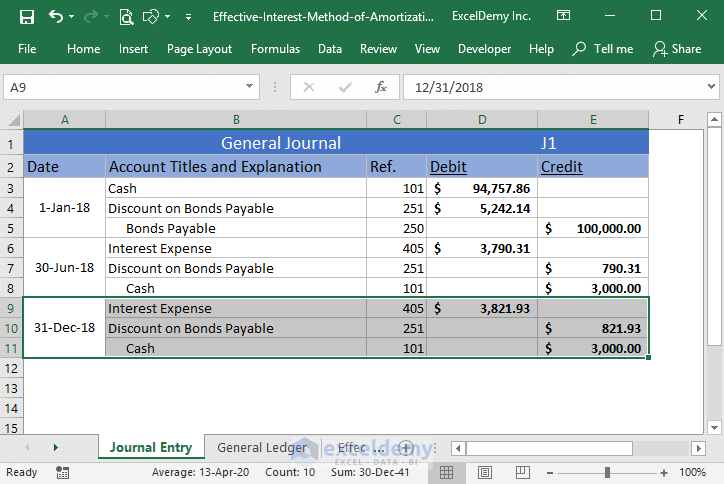Our true cost of fund is: \$95548.17 x (8%/2) = \$3821.93. So, I have debited Interest Expense account with this amount.

The Cash account is credited by \$3000 (paid to bondholder).

And Discount on Bonds Payable account is credited by the unpaid interest = \$3821.93 – \$3000 = \$821.93.

So, our new carrying value of the bond and General Ledger will be like the following image.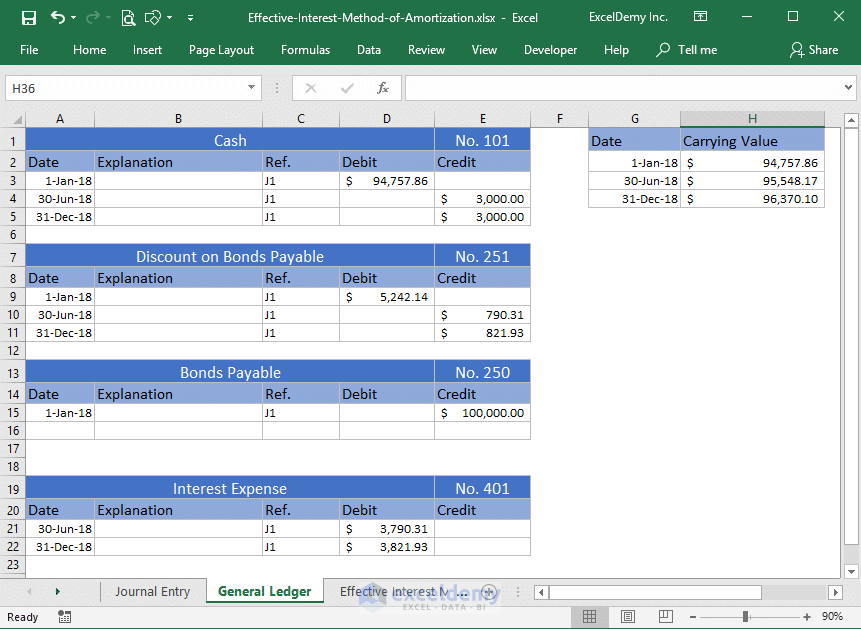### Step 5: Final picture of the General Ledger

At the end of the maturity of the bond, this will be the General Ledger of the bond.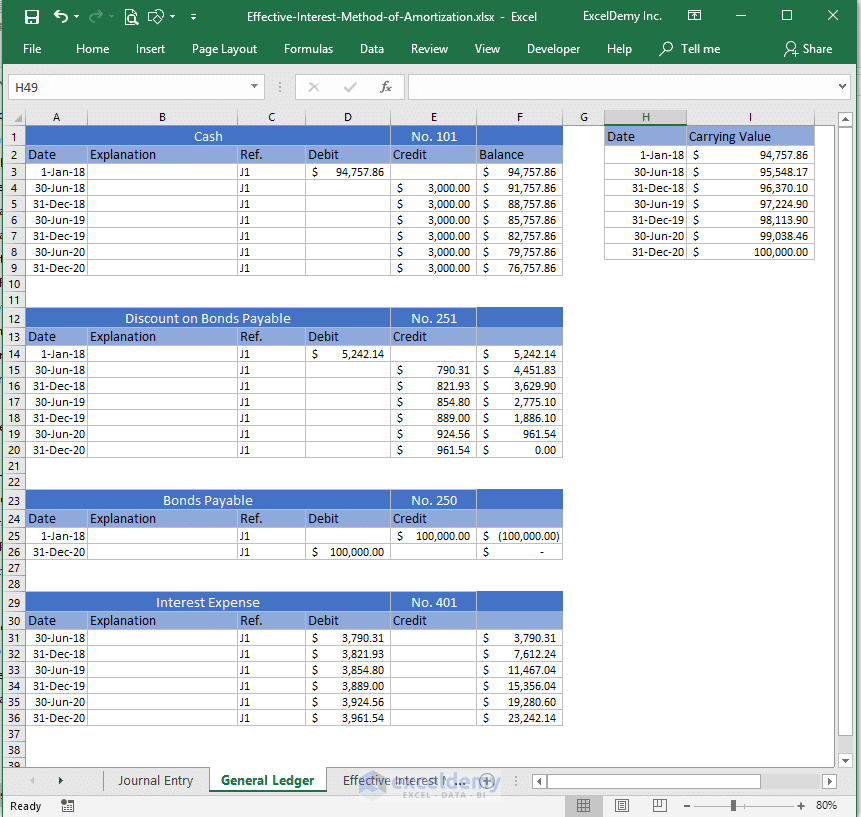Observe this image very carefully. You see that the total cash outflow from your company is: \$76,757.86 – \$100,000 = -\$23,242.14. And this is the total Interest Expense for this bond for 3 years.

### Step 6: The Amortization Table

I hope you have understood the theory part. Making an amortization table is easy if you know the above basics.

The following image shows the Effective Interest Method of Amortization using Excel.If you did understand the theory part, then the above image is self-explanatory. This is just the summary of all the theories I have explained above (step by step).

## Effective Interest Method of Amortization in Excel (For Bonds Sold with Premium)

Here is the amortization table for bonds sold with premium.For bonds that are sold in premium, you have to debit the Premium on Bonds Payable account gradually. It is not a contra liability account. You can call it an adjunct account because its purpose is to balance the additional amount (for this bond: \$102754.06 – \$100000 = \$2754.06) the company receives from the bonds sold on premium.

The carrying value of the bond is calculated by subtracting the additional interest (the company pays to the bondholder) from the current book value.

For example, on Jan 01, 2018, the carrying value of the bond was \$102754.06. On June 30, 2018, the company paid \$3000 to the bondholder. But the genuine cost of the fund (\$102754.06) was \$102754.06 x (5%/2 ) = \$2568.85. So, the company is paying extra of amount: \$3000 – \$2568.85 = \$431.15.

This additional interest is subtracted from the current carrying value of the bond to get the new carrying value: \$102754.06 – \$431.15 = \$102322.91.

## Conclusion

I hope you understood the whole process but if you have any problem to understand anything of this article, let me know in the comment box.

Hello!
Welcome to my Excel blog! It took me some time to be a fan of Excel. But now I am a die-hard fan of MS Excel. I learn new ways of doing things with Excel and share here. Not only how to guide on Excel, but you will get also topics on Finance, Statistics, Data Analysis, and BI. Stay tuned!

1 Comment
1. Replysiti zubaidah Aug 27, 2020 at 2:40 PM

Hi,

I’m trying to calculated amortization using your template but unfortunately i didn’t get the figures.

May I know how to fill the boxes with the information below:-

as example:

Government Bond
Unit : 3,000,000
Coupon Rate : 3.422
Issued Date : 31/03/2020
Maturity Date : 30/09/2027
Purchased Date : 31/03/2020
Settlement Date : 01/04/2020

Purchase Price : 99.934
Purchase YTM : 3.432

Cost : 2,998,032.00
Accured Interest : 280.49
Total Paid : 2,998,312.49

Amortization for end of April Report is (16.42) and I’m trying to get this figures but i failed to get its.